# How to convert 24h format to 12h using the TEXT function

In today's Excel section, I will share with you how to calculate the total time in hours, minutes, seconds and change the 24-hour time format to 12 o'clock and vice versa using the TEXT function.

### #first. How to convert 24h format to 12h using the TEXT function

+ Step 1: First, open the Excel file you need to process.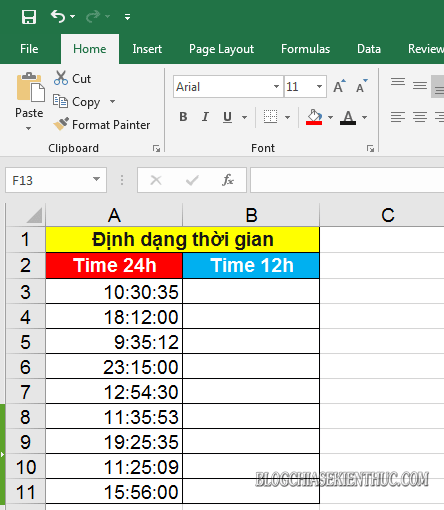+ Step 2: To convert the time format from 24h to 12h, click on the cell where you need to output the value (result) => and enter the formula as follows => and `Enter` to execute:
= TEXT(Choose a time value, ”hh: mm: ss AM / PM")
Inside:
• hh: mm: ss: The value of hours, minutes, seconds respectively.
• AM / PM: Is the format of the morning time AM, afternoon PM.
Applied to the example we get the formula: = TEXT (A3, "hh: mm: ss AM / PM")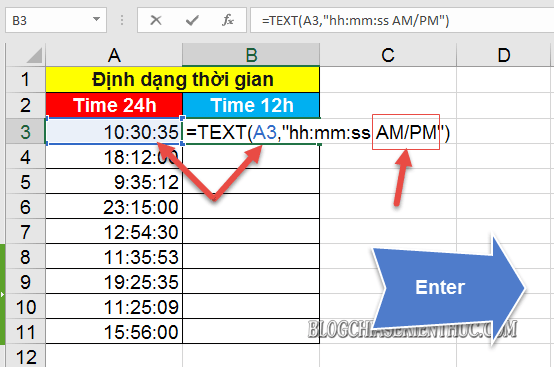+ Step 3: After pressing Enter we will get the results as shown below. Now, you should fill the formula cell down the list to apply to the whole list.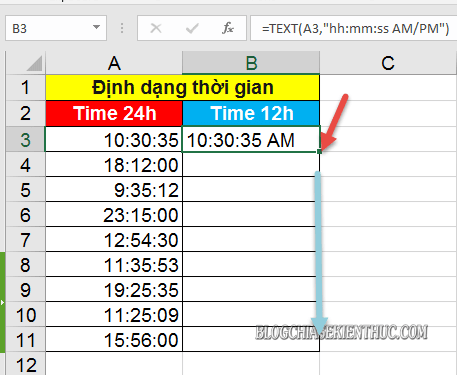Once done, we will have the results format time 12h.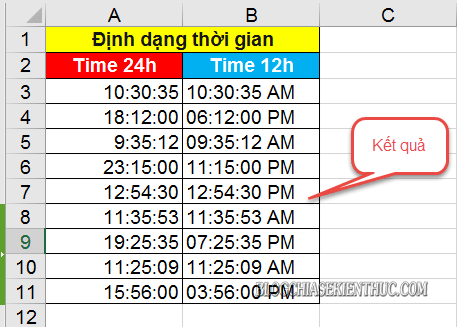### #2. How to convert 12h to 24h format using the TEXT function

With the opposite case if you need convert time from 12h to 24h format then you apply the following formula formula:
= TEXT(worth the time,"Hh: mm: ss")
Applied to the example we have the following function formula: = TEXT (B3, "hh: mm: ss")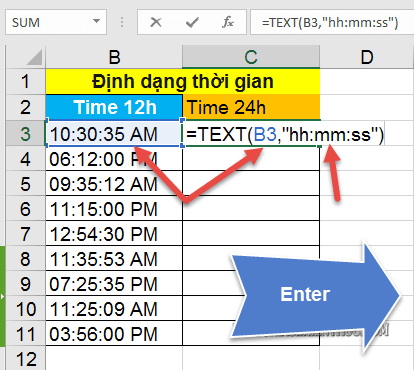Then you Fill the formula cell results down to the remaining cells is done.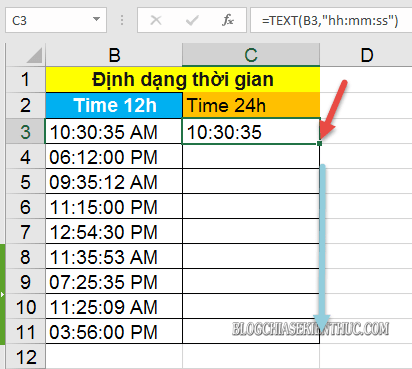The final result, after we are done, will be as shown below.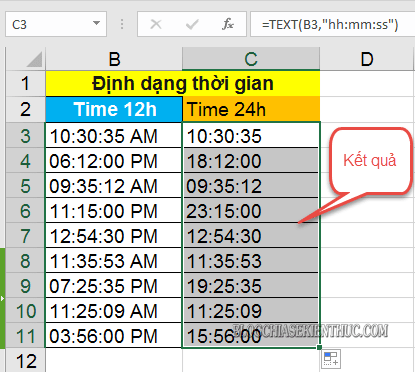### # 3. How to calculate total time hours, minutes, and seconds with the TEXT function

To calculate the time from 0:00:00 to the current time, enter the following formula => and press `Enter` to look up .:
= TEXT(worth the time,"Hh")
Applied to the example we have the following function: = TEXT (C3, "hh")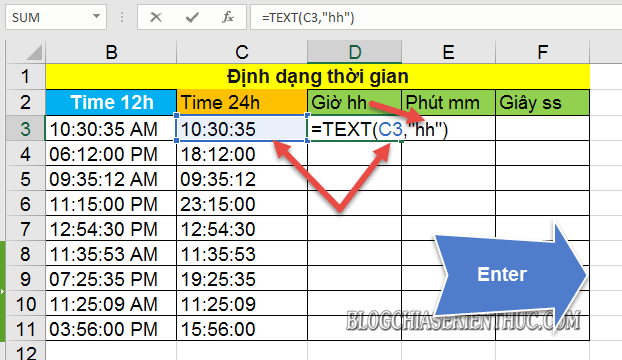****************************************
Similarly, with the total number of minutes you enter the following function:
= TEXT(worth the time, "(Mm)")
Note: To calculate the total number of minutes you add square brackets to the value mm.
Applied to the example we have the function formula: = TEXT (C3, ”(mm)”)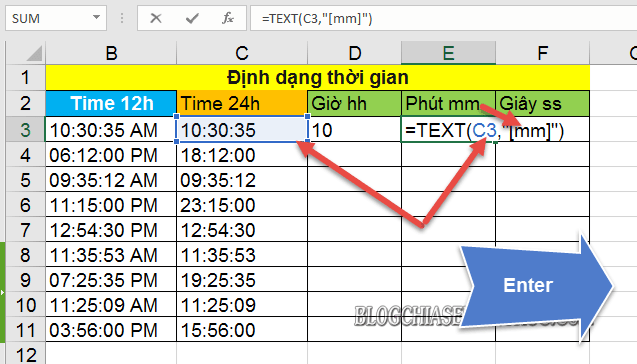****************************************
And with the calculation of the total number of seconds, you also apply the same as the total number of minutes, you use the following formula formula:
= TEXT(worth the time, "(Ss)")
Applied to the example we have the formula: = TEXT (C3, ”(ss)”)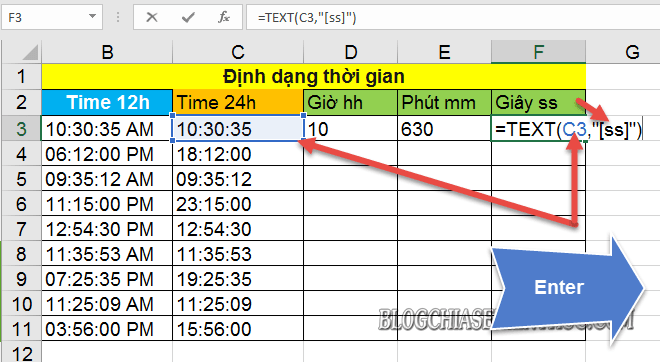Finally, highlight the three values ​​of the hour, minute, and second formulas => and hold the plus sign to fill the whole list.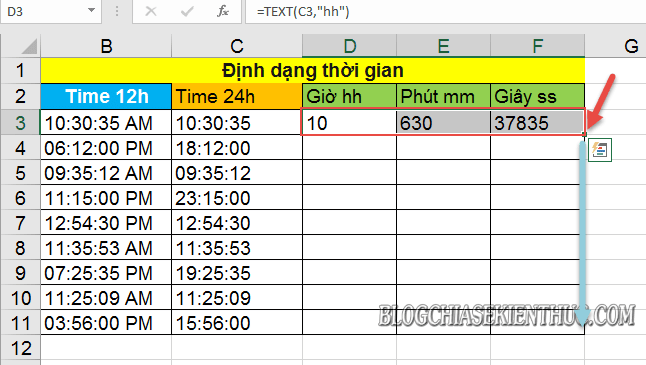And we get the final result as shown below.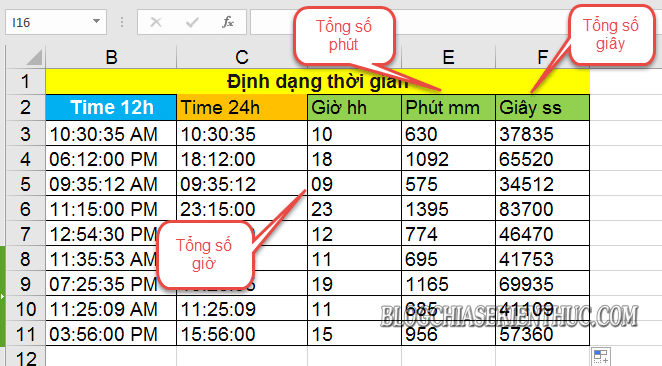### # 4. Epilogue

Okay, so I just gave you very detailed instructions Use the TEXT function to calculate the total number of hours, minutes, and seconds and Change 24h format to 12h in Excel, and vice versa.
In addition, with the TEXT function, you can also calculate indicators such as date, current date, percentage, fraction, or longitude, latitude and currency ...
At this point, my article would like to pause. Hope this tip will be helpful to you.
Good luck !
CTV: Luong Trung